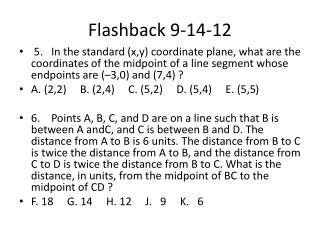DownloadDownload PresentationFlashback 9-14-12

# Flashback 9-14-12

Télécharger la présentation## Flashback 9-14-12

- - - - - - - - - - - - - - - - - - - - - - - - - - - E N D - - - - - - - - - - - - - - - - - - - - - - - - - - -
##### Presentation Transcript

1. Flashback 9-14-12 • 5. In the standard (x,y) coordinate plane, what are the coordinates of the midpoint of a line segment whose endpoints are (–3,0) and (7,4) ? • A. (2,2) B. (2,4) C. (5,2) D. (5,4) E. (5,5) • 6. Points A, B, C, and D are on a line such that B is between A andC, and C is between B and D. The distance from A to B is 6 units. The distance from B to C is twice the distance from A to B, and the distance from C to D is twice the distance from B to C. What is the distance, in units, from the midpoint of BC to the midpoint of CD ? • F. 18 G. 14 H. 12 J. 9 K. 6

2. Joke of the day on a math test: 2+2

3. me: use calculator just in case

4. Multiplication and Division with Mixed Numbers • Change each Mixed Number into an Improper Fraction. • Follow the rules for Multiplying and Dividing Fractions.

5. Multiply or Divide:

6.  Addition and Subtraction with Mixed Numbers • Add the Whole Numbers and then add the Fractions. Simplify. • Subtract the Fractions, then subtract the Whole Numbers. Simplify. • (Borrow, if necessary, if the fraction that you are subtracting is larger than the fraction you are subtracting from.)